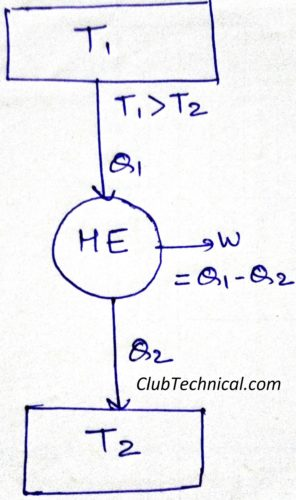# Entropy: How it’s Originated in Thermodynamics [Fully Explained]

By | March 3, 2019

## Birth of Entropy

Entropy is the degree of randomness of a thermodynamic system.

In this article we will discuss how the concept of Entropy is originated in thermodynamics.

Let’s consider a reversible heat engine.From the absolute thermodynamic scale of temperature, we know that.

Q1/Q2 = T1/T2

Q1/T1 – Q2/T2 = 0

Q1/T1 + (-Q2)/T2 = 0

ΣCYCLE (Q/T) = 0

In terms of cyclic integral we can write above equation as

CYCLE (∂QR/T) = 0

We all know that cyclic integral of only a property is zero.

It means ∂QR/T represents a property. Let’s say it dS.

dS = ∂QR/T

Integrating both sides

ΔS = ∫(∂QR/T)

S2 – S1 = ∫(∂QR/T)

We declare this point function ‘S’ as entropy.